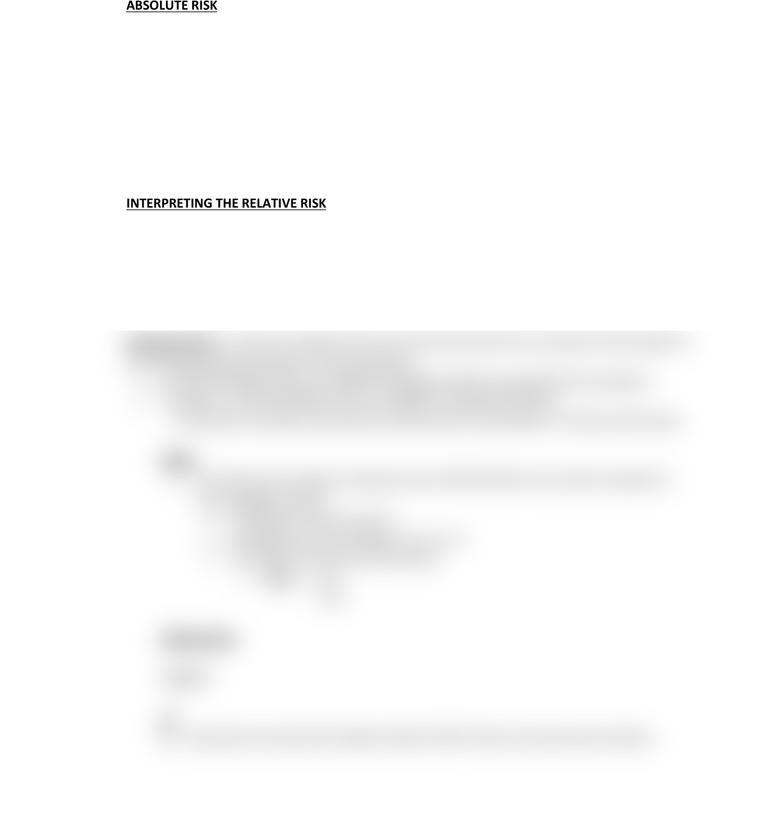Class Notes (1,100,000)
US (440,000)
UC-Irvine (10,000)
PUBHLTH (600)
Lecture 12

# PUBHLTH 101 Lecture Notes - Lecture 12: Odds Ratio, Cohort Study, Relative Risk

Department
Public Health
Course Code
PUBHLTH 101
Professor
David Timberlake
Lecture
12

This preview shows half of the first page. to view the full 2 pages of the document.LEC 12
ABSOLUTE RISK: incidence rate does NOT indicate whether the exposure is associated with an
increased risk of the disease
To address the question of association, we must use approaches that involve explicit
comparisons
Association between a certain exposure & outcome: to measure whether there is an excess
risk of the disease in persons who have been exposed to a certain agent
Possible because participants are randomly assigned/allocated
INTERPRETING THE RELATIVE RISK
RR = 1 Risk in exposed equal to risk in non exposed (no association)
RR > 1 Risk in exposed greater than risk in non exposed (positive association; possible causal
association)
RR < 1 Risk in exposed less than risk in non exposed (negative association; possible protective
association)
Odds Ratio OR: In order to calculate a RR, we must have values for the incidence of the disease in
the exposed and the incidence in the non-exposed
In CASE CONTROL STUDY: we CANNOT calculate incidence in exposed & non-exposed
Therefore, in CASE CONTROL study, we CANNOT calculate RR directly
We have to consider other type of measurement of association in a Case-control study
ODDS
The ratio of the number of ways the event CAN OCCUR to the number of ways the
event CANNOT OCCUR
Probability of event occur: P
Probability of event CANNOT occur: 1 - P
The odds of occurrence of the event:
§ Odds = P
1 - P
ODDS RATIO:
In BOTH